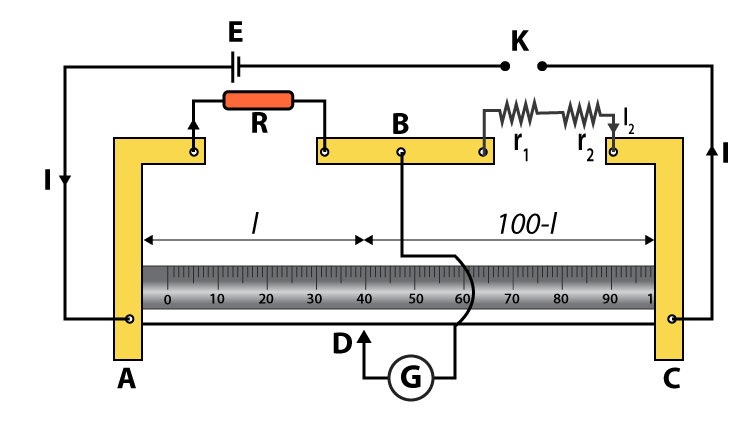# To Verify The Laws Of Combination (Series) Of Resistances Using A Metre Bridge

## Aim

To verify the laws of the combination of resistances using a meter bridge.

## Apparatus/Materials Required

• Meter Bridge
• Leclanche cell
• Resistance Box
• Galvanometer
• Jockey
• Sand Paper
• Connecting wire
• Two resistance wires
• A set square

## Circuit Diagram## Theory

(i) The resistance of a resistance wire or a coil is given by

$$\begin{array}{l}r=\frac{(100-l)}{l}\cdot R\end{array}$$

where R is the resistance from the resistance box in the left gap, and l is the length of the meter bridge wire from zero ends up to the balance point.

(ii) When two resistors r1 and r2 are connected in series, their combined resistance is given as follows:

Rs = r1 + r2

## Procedure

1. Mark the two resistance coils as r1 and r2.
2. To find the value of r1 and r2, follow the same steps as Experiment 1.
3. Connect two coils r1 and r2 in series as shown in the figure.
4. Record the observations as given in the table below

## Observations

 Resistance coil S.No of Observations Resistance from the resistance box Length AD = I cm Length DC = (100 – l) cm Resistance $$\begin{array}{l}r=\frac{(100-l)}{l}\cdot R\end{array}$$ Mean Resistance (ohm) r1 only 1. 2. 3. r1 = …… r2 only 1. 2. 3. r2 = …… r1 and r2 in series 1. 2. 3. Rs = ……

## Result

Within limits of experimental error, theoretical and experimental values of Rs are the same. Hence, the law of resistance in series is verified.

## Viva Voice

1. What is a metre bridge?

Ans:

A metre bridge, also called a slide wire bridge, is an instrument that works on the principle Wheatstone bridge. A meter bridge is used in finding the unknown resistance of a conductor as that of in a Wheatstone bridge

1. How do you find the equivalent resistance of the series combination of resistance?

Ans:

In series combination, the same current passes through each resistor in the chain and the total resistance RT is given by the equation

RT = R1 + R2 + R3 + ……..Rn

Stay tuned with BYJU’S to get the latest notification on CBSE along with CBSE syllabus, sample papers, marking scheme, and more.

## What is the Wheatstone Bridge?Test Your Knowledge On Verify Law Of Combination Of Reistance Using Metre Bridge!